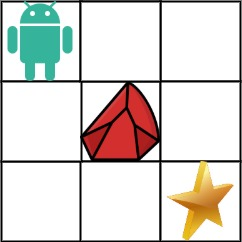엔지니어 게시판
LeetCode 솔루션 분류

# [5/20] 63. Unique Paths II

• mingki 작성
• 작성일

• 413 조회
• 2 댓글

### 본문

[LeetCode 시즌 3] 2022년 5월 20일 문제입니다.

63. Unique Paths II
Medium

You are given an `m x n` integer array `grid`. There is a robot initially located at the top-left corner (i.e., `grid`). The robot tries to move to the bottom-right corner (i.e., `grid[m-1][n-1]`). The robot can only move either down or right at any point in time.

An obstacle and space are marked as `1` or `0` respectively in `grid`. A path that the robot takes cannot include any square that is an obstacle.

Return the number of possible unique paths that the robot can take to reach the bottom-right corner.

The testcases are generated so that the answer will be less than or equal to `2 * 109`.

Example 1:```Input: obstacleGrid = [[0,0,0],[0,1,0],[0,0,0]]
Output: 2
Explanation: There is one obstacle in the middle of the 3x3 grid above.
There are two ways to reach the bottom-right corner:
1. Right -> Right -> Down -> Down
2. Down -> Down -> Right -> Right
```

Example 2:```Input: obstacleGrid = [[0,1],[0,0]]
Output: 1
```

Constraints:

• `m == obstacleGrid.length`
• `n == obstacleGrid[i].length`
• `1 <= m, n <= 100`
• `obstacleGrid[i][j]` is `0` or `1`.

댓글 2

## mingki님의 댓글

• mingki
• 작성일
C++
Runtime: 2 ms, faster than 81.84% of C++ online submissions for Unique Paths II.
Memory Usage: 8 MB, less than 13.17% of C++ online submissions for Unique Paths II.
``````class Solution {
public:
int uniquePathsWithObstacles(vector<vector<int>>& grid) {
int n = grid.size();
int m = grid.size();
vector<vector<int>> dp(n + 1, vector<int>(m + 1, 1));

for (int i = 1; i <= n; ++i) {
dp[i] = grid[i - 1] == 1 ? 0 : dp[i - 1];
}
for (int j = 1; j <= m; ++j) {
dp[j] = grid[j - 1] == 1 ? 0 : dp[j - 1];
}

for (int i = 2; i <= n; ++i) {
for (int j = 2; j <= m; ++j) {
if (grid[i - 1][j - 1] == 0) {
dp[i][j] = dp[i - 1][j] + dp[i][j - 1];
}
else {
dp[i][j] = 0;
}
}
}
return dp[n][m];
}
};``````

## coderoncruise님의 댓글

• coderoncruise
• 작성일
dynamic programming

``````class Solution:
def uniquePathsWithObstacles(self, obstacleGrid: List[List[int]]) -> int:

if obstacleGrid == 1 or obstacleGrid[-1][-1] == 1:
return 0

rows = len(obstacleGrid)
cols = len(obstacleGrid)

board = [ * cols for _ in range(rows)]

for c in range(cols - 1, -1, -1):
if obstacleGrid[-1][c] == 1:
break
board[-1][c] = 1

for r in range(rows - 1, -1 ,-1):
if obstacleGrid[r][-1] == 1:
break
board[r][-1] = 1
print(board)
for r in range(rows - 2, -1, -1):
for c in range(cols - 2, -1, -1):
if obstacleGrid[r][c] == 1:
continue
board[r][c] = board[r+1][c] + board[r][c+1]

return board``````
전체 128 / 1 페이지

### 새댓글

• 등록자 JakeMinSVK 등록일 18:25
• 등록자 JakeMinSVK 등록일 18:24
• 등록자 학부유학생 등록일 06.22
• 등록자 학부유학생 등록일 06.21
• 등록자 Coffee 등록일 06.21
• 등록자 Coffee 등록일 06.21
• 등록자 SVKOREANS 등록일 06.20
• 등록자 학부유학생 등록일 06.20
• 등록자 Coffee 등록일 06.20
• 등록자 키위 등록일 06.20

### Stats

• 현재 접속자 72 명
• 오늘 방문자 654 명
• 어제 방문자 683 명
• 최대 방문자 1,262 명
• 전체 회원수 264 명